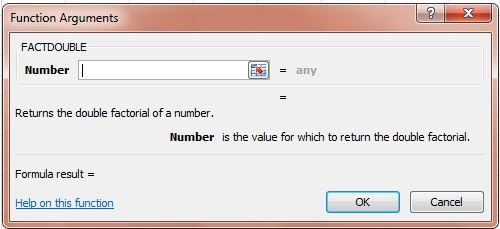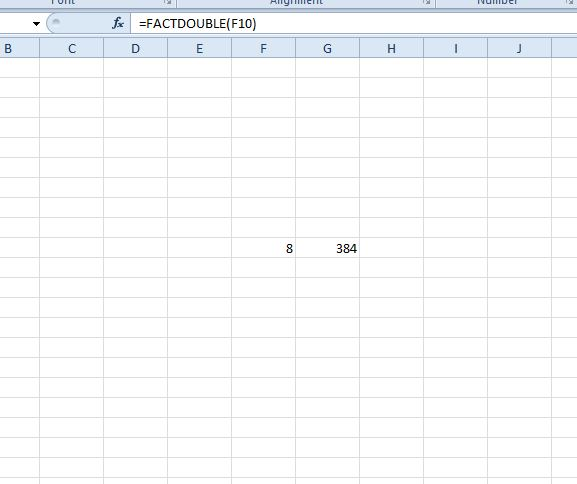# How to use FACTDOUBLE function in Excel spreadsheets [Tip]

This post covered the FACT Excel function that you can find factorials with. You can also find double factorials with Excel 2010/13. The double factorial is the product of all integers from one up to a non-negative integer n with the same parity as n. For example, the double factorial of 8 is 8 x 6 x 4 x 2, which is 384. You can find the double factorial with the FACTDOUBLE function.

For example, enter 8 in an Excel spreadsheet cell. Select a cell to add the function to, and click the fx button beside the formulae bar. Then you should select FACTDOUBLE from the Insert Function window.Then press the button beside the Number field. Select the cell that you entered 8 in. Then press OK to add the FACTDOUBLE function to spreadsheet cell. It will return a value of 384, which is the double factorial of eight.You can also enter this function directly into the formulae bar. Select the bar and enter = FACTDOUBLE. Beside that input the value to find the double factorial for in brackets and press Enter. So the formula could be =FACTDOUBLE(8).